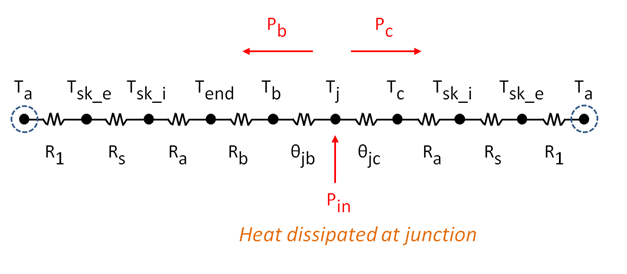# Thermal Resistance Networks: Application

In an earlier blog the thermal resistance network shown in Figure 1 was developed.

Figure 1:The heat dissipation paths from the die are: to the case of the package, and through the BGA into the PCB.  Heat is then dissipated through the skin of the device by convection to the environment. The two dissipation paths are represented by a case resistance branch:

Rcase_branch = θjc+ Ra+ Rs+ R1

And a board resistance branch:

Rboard_branch = R1+Rs+Ra+Rbjb

The total resistance, Rtot , is given by:

And the relationship   q = ΔT / Rtot  relates the entire network resistance, Rtot, to ΔT.

As an example let’s examine the design of a device where power of 1 Watt is used and it is required that a junction temperature, Tj, remain below 120 ⁰C.  If the ambient temperature near the device (Ta) may be 60 ⁰C, a temperature rise (Tj-Ta) of 60 ⁰C is the maximum allowable. Estimates of thermal resistance can be made by describing convection as a resistance using Rconvec = 1/(h*A)  and conduction as a resistance using Rconduc = L/(k*A). The device is a small enclosure, little natural convection can occur due to the proximity of the boundaries, thus convection from the case to the air can be expressed as the limiting case of conduction through an air gap, Ra= Lair/(kair*Acase).   The temperature distribution along the board is not uniform, thus we include conduction driven by convection from the board. The in-plane PCB thermal conductivity is modeled using effective, or volume-averaged, values. Using typical package dimensions, material properties and the package thermal parameters θjc = 1.0 ⁰C/W and θjb = 5.0 ⁰C/W, the calculated thermal resistances for a system level analysis are given below for 2 cases: one with an air gap and second with the air gap filled with thermally conductive paste.

 Name Description Formula With air gap, ⁰C/W With gap filler and conductive plastic, ⁰C/W R1 Convection external to device 1/(hext_nat_conv * Acase_conv) 780 65 Rs Conduction through device skin Lskin_thick/(kplastic*Acase) 3.9 0.039 Ra Conduction through air gap L_air / (k_air * Acase) 88 2.0 Rb Conduction along PCB ((Lb-Lpckg)/2)/(kxy * Ax-sect_b) 33 33

With the air gap a junction temperature of ~505⁰C is predicted, which, even though conservative, is clearly unacceptable. To overcome the problem two options can be considered:

1. Fill the air gap over the component case with thermal gap filler
2. Use thermally conductive plastic as the enclosure material for the device skin, instead of polycarbonate plastic

With these design changes the junction temperature falls to ~101 ⁰C thus providing an operating condition within the target of 120 ⁰C without compromising power demands.